## Sunday, June 16, 2013

### Support for IN CAML operation: Camlex 3.5 and Camlex.Client 1.3 are released

I have good news for Sharepoint developers who use Camlex in their project: I’ve released new versions of Camlex and Camlex.Client which now support IN operation (finally). At first I will show examples and describe some technical details and then add several notes about using of Camlex in different Sharepoint versions (2007, 2010 and 2013).

What is IN operation? As msdn article says:

Specifies whether the value of a list item for the field specified by the FieldRef element is equal to one of the values specified by the Values element.

E.g. if we need to get all list items which have integer Count field in range 0..9 we can use the following query:

`   1: <Where>`
`   2:   <In>`
`   3:     <FieldRef Name="Count" />`
`   4:     <Values>`
`   5:       <Value Type="Integer">0</Value>`
`   6:       <Value Type="Integer">1</Value>`
`   7:       <Value Type="Integer">2</Value>`
`   8:       <Value Type="Integer">3</Value>`
`   9:       <Value Type="Integer">4</Value>`
`  10:       <Value Type="Integer">5</Value>`
`  11:       <Value Type="Integer">6</Value>`
`  12:       <Value Type="Integer">7</Value>`
`  13:       <Value Type="Integer">8</Value>`
`  14:       <Value Type="Integer">9</Value>`
`  15:     </Values>`
`  16:   </In>`
`  17: </Where>`

It was possible to combine multiple Eq operations using Or and get the same result. And with Camlex it was quite easy to do it using single line of code (for better formatting I put it to 3 lines):

`   1:  `
`   2:  `
`   3:  `
`   4: string caml = Camlex.Query().WhereAny(`
`   5:     Enumerable.Range(0, 9).Select<int, Expression<Func<SPListItem, bool>>>(`
`   6:         i => x => (int) x["Count"] == i)).ToString();`

But let’s see the resulting CAML of the above code:

`   1: <Where>`
`   2:   <Or>`
`   3:     <Or>`
`   4:       <Or>`
`   5:         <Or>`
`   6:           <Or>`
`   7:             <Or>`
`   8:               <Or>`
`   9:                 <Or>`
`  10:                   <Eq>`
`  11:                     <FieldRef Name="Count" />`
`  12:                     <Value Type="Integer">0</Value>`
`  13:                   </Eq>`
`  14:                   <Eq>`
`  15:                     <FieldRef Name="Count" />`
`  16:                     <Value Type="Integer">1</Value>`
`  17:                   </Eq>`
`  18:                 </Or>`
`  19:                 <Eq>`
`  20:                   <FieldRef Name="Count" />`
`  21:                   <Value Type="Integer">2</Value>`
`  22:                 </Eq>`
`  23:               </Or>`
`  24:               <Eq>`
`  25:                 <FieldRef Name="Count" />`
`  26:                 <Value Type="Integer">3</Value>`
`  27:               </Eq>`
`  28:             </Or>`
`  29:             <Eq>`
`  30:               <FieldRef Name="Count" />`
`  31:               <Value Type="Integer">4</Value>`
`  32:             </Eq>`
`  33:           </Or>`
`  34:           <Eq>`
`  35:             <FieldRef Name="Count" />`
`  36:             <Value Type="Integer">5</Value>`
`  37:           </Eq>`
`  38:         </Or>`
`  39:         <Eq>`
`  40:           <FieldRef Name="Count" />`
`  41:           <Value Type="Integer">6</Value>`
`  42:         </Eq>`
`  43:       </Or>`
`  44:       <Eq>`
`  45:         <FieldRef Name="Count" />`
`  46:         <Value Type="Integer">7</Value>`
`  47:       </Eq>`
`  48:     </Or>`
`  49:     <Eq>`
`  50:       <FieldRef Name="Count" />`
`  51:       <Value Type="Integer">8</Value>`
`  52:     </Eq>`
`  53:   </Or>`
`  54: </Where>`

Comparing with 1st example, it looks not very nice. If it doesn’t matter for you, you still can use OR syntax. However now it is possible to write simpler expression which will produce IN syntax:

`   1: string caml =`
`   2:     Camlex.Query().Where(x => Enumerable.Range(0, 9).Contains((int)x["Count"]))`
`   3:         .ToString();`

It will produce CAML which we already saw:

`   1: <Where>`
`   2:   <In>`
`   3:     <FieldRef Name="Count" />`
`   4:     <Values>`
`   5:       <Value Type="Integer">0</Value>`
`   6:       <Value Type="Integer">1</Value>`
`   7:       <Value Type="Integer">2</Value>`
`   8:       <Value Type="Integer">3</Value>`
`   9:       <Value Type="Integer">4</Value>`
`  10:       <Value Type="Integer">5</Value>`
`  11:       <Value Type="Integer">6</Value>`
`  12:       <Value Type="Integer">7</Value>`
`  13:       <Value Type="Integer">8</Value>`
`  14:     </Values>`
`  15:   </In>`
`  16: </Where>`

For those who worked with NHibernate or Linq2Sql this syntax won’t be new: these ORM frameworks use the same syntax for generating of SQL queries with IN operator. I intentionally showed example with dynamically populated array (Enumerable.Range(0, 9)): code supports any expression which produces IEnumerable:

`   1: var caml = Camlex.Query().Where(x => getArray().Contains((int)x["Count"])).ToString();`
`   2: ...`
`   3: List<int> getArray()`
`   4: {`
`   5:     var list = new List<int>();`
`   6:     for (int i = 0; i < 10; i++)`
`   7:     {`
`   8:         list.Add(i);`
`   9:     }`
`  10:     return list;`
`  11: }`

(Do you see the difference between the last example which function which returns List<int>, and previous example, which uses IEnumerable? If yes, you know C# well :). Class List<T> has own Contains method, while for IEnumerable<int> from first example Linq Contains extension method was used. As you can see Camlex may work with both of them. Note that if you use Linq you should add “using System.Linq;” to your file).

I.e. basic syntax is the following:

`   1: var caml = Camlex.Query().Where(x =>`
`   2:     enumerable.Contains((Type)x["FieldTitle"])).ToString();`

Of course you may write array with constants:

`   1: string c = Camlex.Query().Where(x => new[] {0, 1, 2, 3, 4, 5, 6, 7, 8, 9}`
`   2:     .Contains((int) x["Count"])).ToString();`

Also it will work with any type supported for Value element:

`   1: string c = Camlex.Query().Where(x => new[]`
`   2:     {"zero", "one", "two", "three", "four", "five", "six", "seven", "eight", "nine"}`
`   3:         .Contains((string) x["Title"])).ToString();`

It will produce the following result:

`   1: <Where>`
`   2:   <In>`
`   3:     <FieldRef Name="Title" />`
`   4:     <Values>`
`   5:       <Value Type="Text">zero</Value>`
`   6:       <Value Type="Text">one</Value>`
`   7:       <Value Type="Text">two</Value>`
`   8:       <Value Type="Text">three</Value>`
`   9:       <Value Type="Text">four</Value>`
`  10:       <Value Type="Text">five</Value>`
`  11:       <Value Type="Text">six</Value>`
`  12:       <Value Type="Text">seven</Value>`
`  13:       <Value Type="Text">eight</Value>`
`  14:       <Value Type="Text">nine</Value>`
`  15:     </Values>`
`  16:   </In>`
`  17: </Where>`

Now it uses Text type in Value elements.

That was only half of the story. If you follow the Camlex, you probably know that starting with version 3.0 it became bidirectional (I wrote about it here: Camlex.NET became bidirectional and goes online. Version 3.0 is released). So all conversions are added with 2 directions: from expression to CAML and from CAML to expression:

`   1: var xml =`
`   2:     "<Query>" +`
`   3:     "  <Where>" +`
`   4:     "    <In>" +`
`   5:     "      <FieldRef Name=\"Title\" />" +`
`   6:     "      <Values>" +`
`   7:     "        <Value Type=\"Text\">zero</Value>" +`
`   8:     "        <Value Type=\"Text\">one</Value>" +`
`   9:     "        <Value Type=\"Text\">two</Value>" +`
`  10:     "        <Value Type=\"Text\">three</Value>" +`
`  11:     "        <Value Type=\"Text\">four</Value>" +`
`  12:     "        <Value Type=\"Text\">five</Value>" +`
`  13:     "        <Value Type=\"Text\">six</Value>" +`
`  14:     "        <Value Type=\"Text\">seven</Value>" +`
`  15:     "        <Value Type=\"Text\">eight</Value>" +`
`  16:     "        <Value Type=\"Text\">nine</Value>" +`
`  17:     "      </Values>" +`
`  18:     "    </In>" +`
`  19:     "  </Where>" +`
`  20:     "</Query>";`
`  21:  `
`  22: var expr = Camlex.QueryFromString(xml).ToExpression();`

Applications of that feature:

1. on http://camlex-online.org/ add xml shown below to the textarea and click Convert to C#. It will show you how this query will be produced by Camlex:

`   1: Camlex.Query().Where(x => new[] {`
`   2:     "zero", "one", "two", "three", "four", "five", "six", "seven", "eight", "nine" }`
`   3:         .Contains((string)x["Title"]))`

2. it is possible to add more conditions to the existing IN query which is stored as xml, or add IN query to existing query:

`   1: var xml =`
`   2:     "<Query>" +`
`   3:     "  <Where>" +`
`   4:     "    <In>" +`
`   5:     "      <FieldRef Name=\"Title\" />" +`
`   6:     "      <Values>" +`
`   7:     "        <Value Type=\"Text\">one</Value>" +`
`   8:     "        <Value Type=\"Text\">two</Value>" +`
`   9:     "      </Values>" +`
`  10:     "    </In>" +`
`  11:     "  </Where>" +`
`  12:     "</Query>";`
`  13:  `
`  14: string caml = Camlex.Query().WhereAll(xml, x => (int)x["Count"] == 1).ToString();`

will produce:

`   1: <Where>`
`   2:   <And>`
`   3:     <Eq>`
`   4:       <FieldRef Name="Count" />`
`   5:       <Value Type="Integer">1</Value>`
`   6:     </Eq>`
`   7:     <In>`
`   8:       <FieldRef Name="Title" />`
`   9:       <Values>`
`  10:         <Value Type="Text">one</Value>`
`  11:         <Value Type="Text">two</Value>`
`  12:       </Values>`
`  13:     </In>`
`  14:   </And>`
`  15: </Where>`

I.e. Camlex may combine several string queries using Or and And, or even mix string query with expression as shown above. This feature was added in previous releases.

That was part with examples. Now about using of Camlex in different Sharepoint versions. Camlex was initially developed for Sharepoint 2007 and it references Microsoft.SharePoint.dll of 12.0.0.0 version (it only references it for compilation. Camlex doesn’t call any methods of Sharepoint API). However thanks to assemblies binding redirect in Sharepoint it works also in 2010 and 2013 (see this post for details why: Assembly binding redirect in Sharepoint 2010: how old code for SP 2007 works in SP 2010). IN CAML operation was introduced with Sharepoint 2010, i.e. it won’t work in Sharepoint 2007. So before to release new version 3.5 with IN operation support I choose between 2 options:

1. add it to existing Camlex and continue to use Microsoft.SharePoint.dll of 12.0.0.0 version.
Pros: one dll which works in all Sharepoint versions, it is simpler for developers who will want to install it.
Cons: IN element will be created now also for Sharepoint 2007, but it won’t work when try to execute this query against data.

2. create separate branch for Sharepoint 2007 and leave reference to 12.0.0.0 assembly only there, while in default branch change it to 14.0.0.0, so it will be used only starting with Sharepoint 2010, where IN operaion is supported.
Pros: each branch will have only supported features in appropriate version.
Cons: developers should choose correct version by themselves, codeplex is not very good in showing them, it is quite simple to get lost in releases there.

Also with 2nd option it would be necessary to maintain one more branch (currently there are 2 branches: default for basic Camlex and client branch for Camlex for client object model. BTW Camlex.Client.dll of version 1.3 supports IN operator as well). As currently I’m the only continuous contributor of the project and I have very limited time, I choose option 1 for now. May be in future I will separate branches, but for a while there will be single Camlex branch which will work with all Sharepoint versions, but generated CAML may not work in Sharepoint 2007.

With codeplex assemblies I also updated nuget packages. You can install latest versions by the following commands:

basic Camlex:

`   1: Install-Package Camlex.NET.dll`

for client object model:

`   1: Install-Package Camlex.Client.dll`

In my next plans to add rest of CAML elements introduced with Sharepoint 2010 (Include, NotInclude) and Joins. They also should be added of course at some point of course.

1.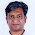You have a new fan!

2.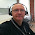Broken in the v4.0. Please check.

3.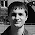Dmitry, could you post example of the expression which doesn't work? All unit tests from previous versions were green in 4.0 version.

4.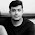Alexey, i need ur help!!!
http://stackoverflow.com/questions/31393887/wrong-results-with-caml-query

5.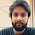How we can use in query with lookup ids

6.Nikhil,
check here: http://sharepoint.stackexchange.com/questions/50283/how-to-combine-in-opeator-with-lookupids-in-a-caml-query.